Smartick is a fun way to learn math!Aug29

# Examples of Equivalent Fractions

Welcome back to the Smartick blog! Do you know what equivalent fractions are? After reading this post with examples of equivalent fractions, you’ll be an expert.

Equivalent fractions are fractions that represent the same amount, even though they may appear to be different.

Let’s look at this example: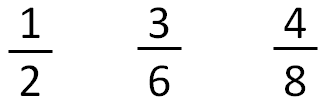Do you think these fractions represent the same amount, or are they all different amounts?

Let’s look at this with the following pictures:

• We divide the cake into the number of slices indicated by the denominator.
• We take as many slices (the colored portion) as indicated by the numerator.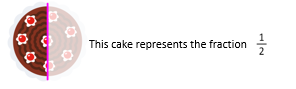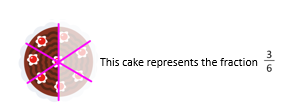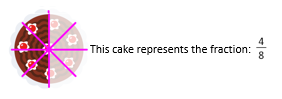The three fractions are equivalent fractions because they represent the same amount of cake, half of it in this case.

How can we know if two fractions are equivalent? By cross-multiplication.

This method consists of cross-multiplying the two fractions: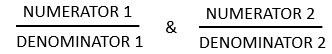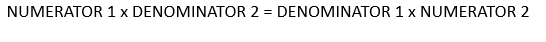Are the following equivalent fractions?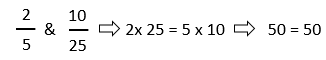YES, they are equivalent fractions

Are the following equivalent fractions?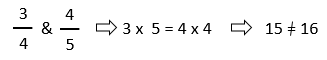In this case, NO, they are not equivalent fractions.

Remember that in order to learn everything about equivalent fractions and all other elementary math topics, the best thing to do is to sign up with Smartick right now and try it for free!

Fun is our brain’s favorite way of learning
Diane Ackerman
Smartick is a fun way to learn math
• 15 fun minutes a day